## RD Sharma Class 9 Solutions Chapter 4 Algebraic Identities Ex 4.3

These Solutions are part of RD Sharma Class 9 Solutions. Here we have given RD Sharma Class 9 Solutions Chapter 4 Algebraic Identities Ex 4.3

Question 1.
Find the cube of each of the following binomial expressions: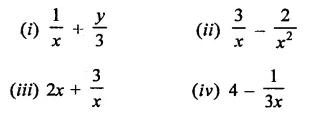Solution: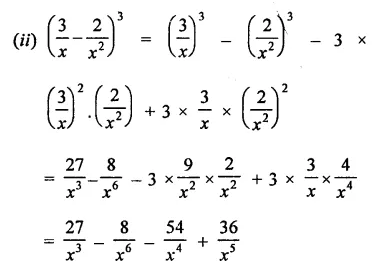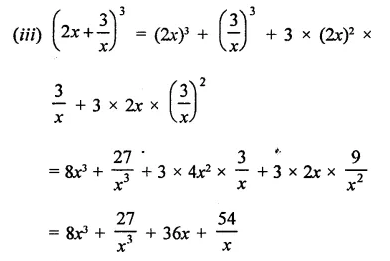Question 2.
If a + b = 10 and ab = 21, find the value of a3 + b3.
Solution:
a + b = 10, ab = 21
Cubing both sides,
(a + b)3 = (10)3
⇒ a3 + 63 + 3ab (a + b) = 1000
⇒  a3 + b3 + 3 x 21 x 10 = 1000
⇒  a3 + b3 + 630 = 1000
⇒  a3 + b3 = 1000 – 630 = 370
∴ a3 + b3 = 370

Question 3.
If a – b = 4 and ab = 21, find the value of a3-b3.
Solution:
a – b = 4, ab= 21
Cubing both sides,
⇒ (a – A)3 = (4)3
⇒ a3 – b3 – 3ab (a – b) = 64
⇒ a3-i3-3×21 x4 = 64
⇒  a3 – 63 – 252 = 64
⇒  a3 – 63 = 64 + 252 =316
∴ a3 – b3 = 316

Question 4.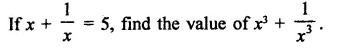Solution: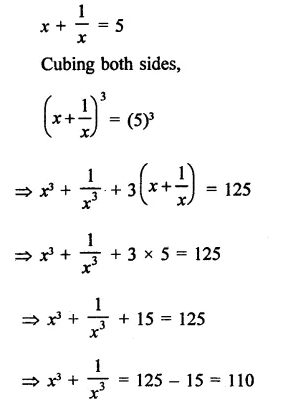Question 5.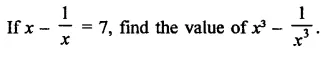Solution: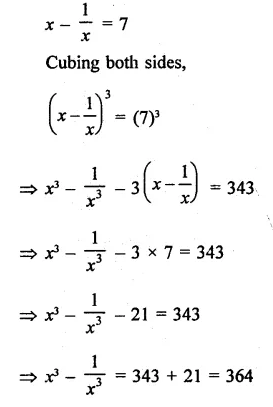Question 6.Solution:Question 7.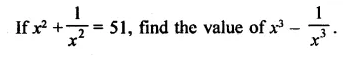Solution: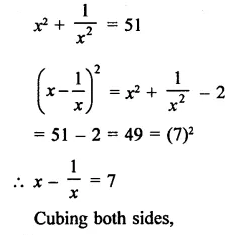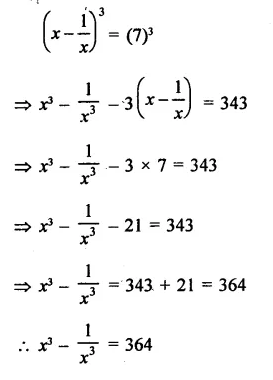Question 8.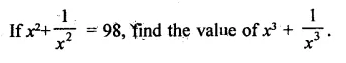Solution: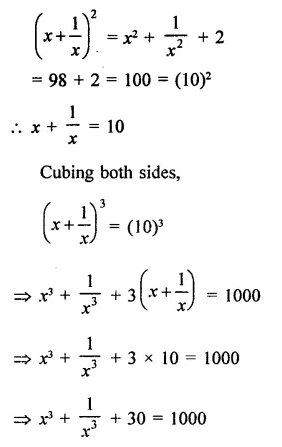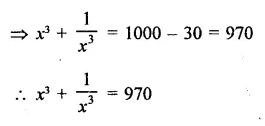Question 9.
If 2x + 3y = 13 and xy = 6, find the value of 8x3 + 21y3.
Solution:
2x + 3y = 13, xy = 6
Cubing both sides,
(2x + 3y)3 = (13)3
⇒ (2x)3 + (3y)3 + 3 x 2x x 3X2x + 3y) = 2197
⇒ 8x3 + 27y3 + 18xy(2x + 3y) = 2197
⇒ 8x3 + 27y3 + 18 x 6 x 13 = 2197
⇒ 8X3 + 27y3 + 1404 = 2197
⇒  8x3 + 27y3 = 2197 – 1404 = 793
∴ 8x3 + 27y3 = 793

Question 10.
If 3x – 2y= 11 and xy = 12, find the value of 27x3 – 8y3.
Solution:
3x – 2y = 11 and xy = 12 Cubing both sides,
(3x – 2y)3 = (11)3
⇒  (3x)3 – (2y)3 – 3 x 3x x 2y(3x – 2y) =1331
⇒  27x3 – 8y3 – 18xy(3x -2y) =1331
⇒   27x3 – 8y3 – 18 x 12 x 11 = 1331
⇒  27x3 – 8y3 – 2376 = 1331
⇒  27X3 – 8y3 = 1331 + 2376 = 3707
∴ 2x3 – 8y3 = 3707

Question 11.
Evaluate each of the following:
(i)  (103)3
(ii) (98)3
(iii) (9.9)3
(iv) (10.4)3
(v) (598)3
(vi) (99)3
Solution:
We know that (a + bf = a3 + b3 + 3ab(a + b) and (a – b)3= a3 – b3 – 3 ab(a – b)
Therefore,
(i)  (103)3 = (100 + 3)3
= (100)3 + (3)3 + 3 x 100 x 3(100 + 3)    {∵ (a + b)3 = a3 + b3 + 3ab(a + b)}
= 1000000 + 27 + 900 x 103
= 1000000 + 27 + 92700
= 1092727
(ii) (98)3 = (100 – 2)3
= (100)3 – (2)3 – 3 x 100 x 2(100 – 2)
= 1000000 – 8 – 600 x 98
= 1000000 – 8 – 58800
= 1000000-58808
= 941192
(iii) (9.9)3 = (10 – 0.1)3
= (10)3 – (0.1)3 – 3 X 10 X 0.1(10 – 0.1)
= 1000 – 0.001 – 3 x 9.9
= 1000 – 0.001 – 29.7
= 1000 – 29.701
= 970.299
(iv) (10.4)3 = (10 + 0.4)3
= (10)3 + (0.4)3 + 3 x 10 x 0.4(10 + 0.4)
= 1000 + 0.064 + 12(10.4)
= 1000 + 0.064 + 124.8 = 1124.864
(v) (598)3 = (600 – 2)3
= (600)3 – (2)3 – 3 x 600 x 2 x (600 – 2)
= 216000000 – 8 – 3600 x 598
= 216000000 – 8 – 2152800
= 216000000 – 2152808
= 213847192
(vi) (99)3 = (100 – 1)3
= (100)3 – (1)3 – 3 x 100 x 1 x (100 – 1)
= 1000000 – 1 – 300 x 99
= 1000000 – 1 – 29700
= 1000000 – 29701
= 970299

Question 12.
Evaluate each of the following:
(i)  1113 – 893
(ii) 463 + 343
(iii) 1043 + 963
(iv) 933 – 1073
Solution:
We know that a3 + b3 = (a + bf – 3ab(a + b) and a3 – b3 = (a – bf + 3 ab(a – b)
(i) 1113 – 893
= (111 – 89)3 + 3 x ill x 89(111 – 89)
= (22)3 + 3 x 111 x 89 x 22
= 10648 + 652014 = 662662
(OR)
(a + b)3 – (a – b)3 = 2(b3 + 3a2b)
= 1113 – 893 = (100 + 11)3 – (100 – 11)3
= 2(113 + 3 x 1002 x 11]
= 2(1331 + 330000]
= 331331 x 2 = 662662
(a + b)3 + (a- b)3 = 2(b3 + 3ab2)
(ii) 463 + 343 = (40 + 6)3 + (40 – 6)3
= 2[(40)3 + 3 x 40 x 62]
= 2[64000 + 3 x 40 x 36]
= 2[64000 + 4320]
= 2 x 68320 = 136640
(iii) 1043 + 963 = (100 + 4)3 + (100 – 96)3
= 2 [a3 + 3 ab2]
= 2[(100)3 + 3 x 100 x (4)2]
= 2[ 1000000 + 300 x 16]
= 2[ 1000000 + 4800]
= 1004800 x 2 = 2009600
(iv) 933 – 1073 = -[(107)3 – (93)3]
= -[(100 + If – (100 – 7)3]
= -2[b3 + 3a2b)]
= -2[(7)3 + 3(100)2 x 7]
= -2(343 + 3 x 10000 x 7]
= -2[343 + 210000]
= -2 = -420686

Question 13.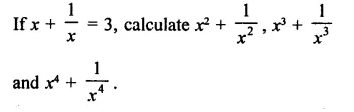Solution: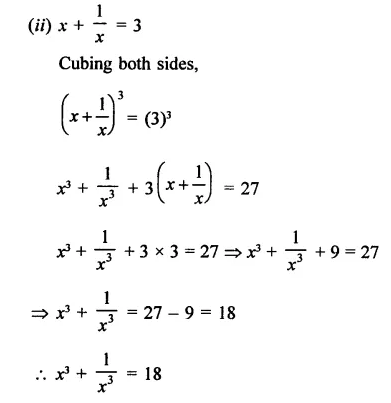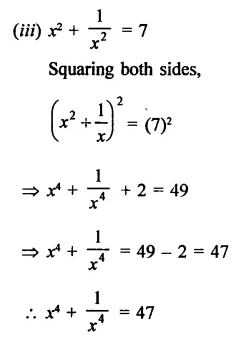Question 14.
Find the value of 27X3 + 8y3 if
(i) 3x + 2y = 14 and xy = 8
(ii) 3x + 2y = 20 and xy = $$\frac { 14 }{ 9 }$$
Solution: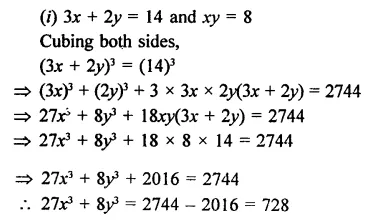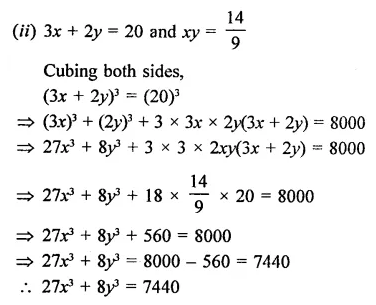Question 15.
Find the value of 64x3 – 125z3, if 4x – 5z = 16 and xz = 12.
Solution:
4x – 5z = 16, xz = 12
Cubing both sides,
(4x – 5z)3 = (16)3
⇒ (4x)3 – (5y)3 – 3 x 4x x 5z(4x – 5z) = 4096
⇒ 64x3 – 125z3 – 3 x 4 x 5 x xz(4x – 5z) = 4096
⇒  64x3 – 125z3 – 60 x 12 x 16 = 4096
⇒ 64x3 – 125z3 – 11520 = 4096
⇒  64x3 – 125z3 = 4096 + 11520 = 15616

Question 16.Solution:Question 17.
Simplify each of the following: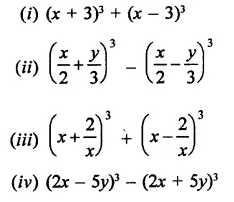Solution: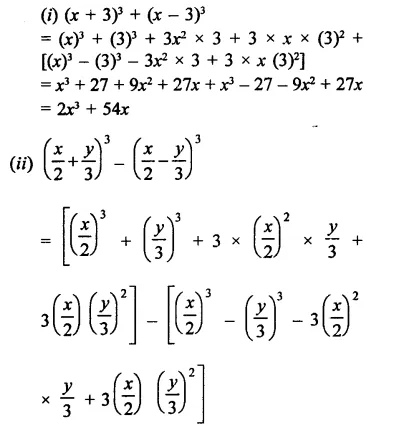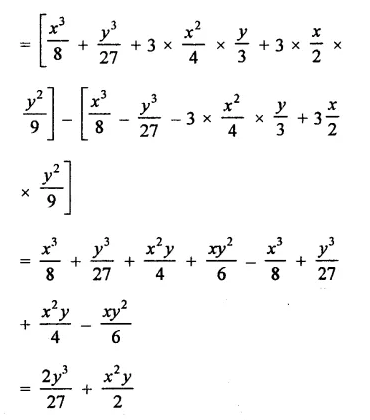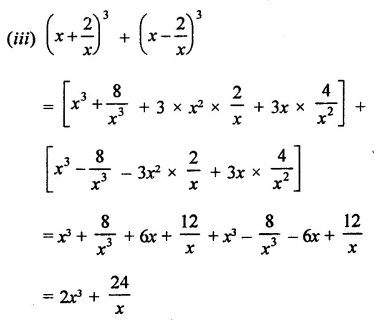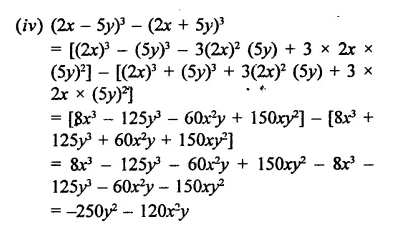Question 18.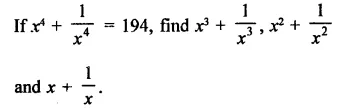Solution: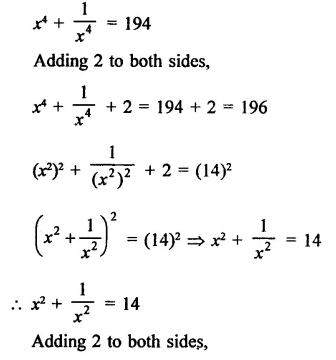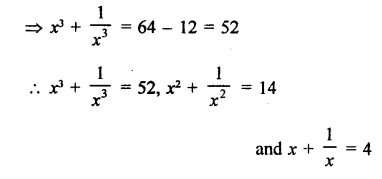Question 19.Solution: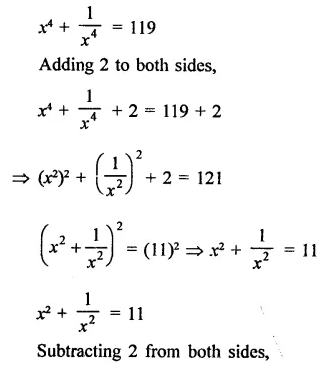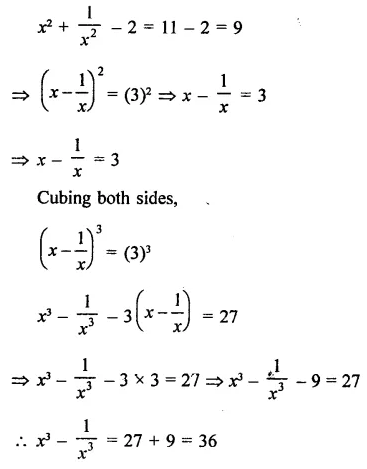Hope given RD Sharma Class 9 Solutions Chapter 4 Algebraic Identities Ex 4.3 are helpful to complete your math homework.

If you have any doubts, please comment below. Learn Insta try to provide online math tutoring for you.